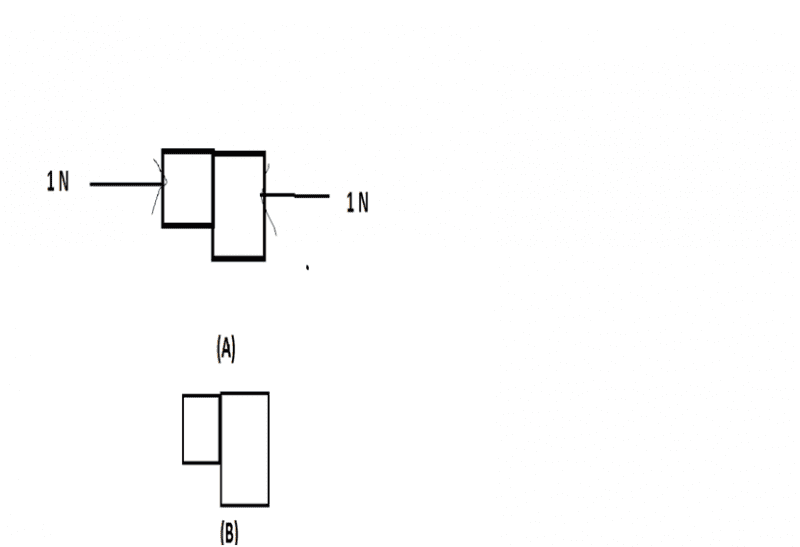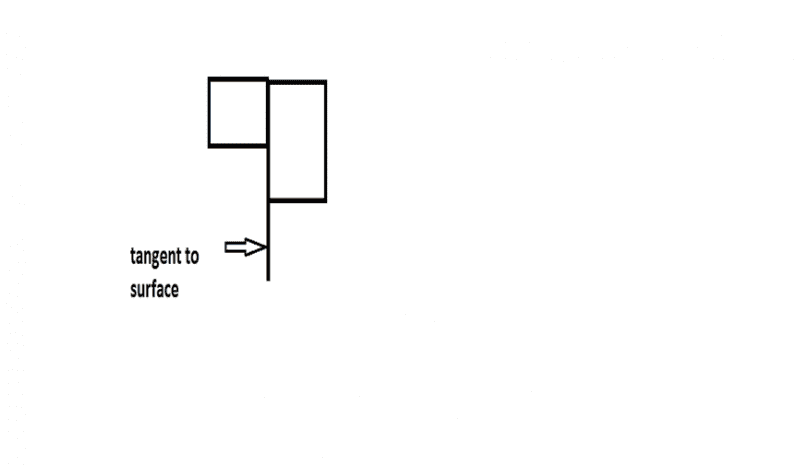# Normal contact force

gracyMy teacher says
In case (A) there would be normal contact force between the blocks.But in case (B)There will not be any normal contact force between the blocks.
I am really not getting this.I think The normal force is the support force exerted upon an object that is in contact with another stable object. For example, if a book is resting upon a surface, then the surface is exerting an upward force upon the book in order to support the weight of the book.But my teacher says the objects should compress each other,deform each other then only there would be normal contact force between them.

## Answers and Replies

Mentor
You're both right. When the book is sitting on the desk at equilibrium, the book has been slightly deformed by the normal stress exerted by the desk, and the region of the desk in close proximity to the book has been slightly deformed by the normal stress exerted by the book. This just acknowledges the fact that, when you exert a force on a non-rigid body, it can deform.

Chet

Homework Helper
Gold Member
View attachment 80931
My teacher says
In case (A) there would be normal contact force between the blocks.But in case (B)There will not be any normal contact force between the blocks.
I am really not getting this.I think The normal force is the support force exerted upon an object that is in contact with another stable object. For example, if a book is resting upon a surface, then the surface is exerting an upward force upon the book in order to support the weight of the book.But my teacher says the objects should compress each other,deform each other then only there would be normal contact force between them.
I agree with your teacher. In case B, if there was a normal contact force of one block on the other, the blocks would accelerate horizontally because there would be a net horizontal force acting (in a free body diagram of one block). This is not the case; there might be friction between the 2 acting downward, but there is no force acting normal to the surface, even though they are marginally touching (barely) each other.

gracy
When the book is sitting on the desk at equilibrium, the book has been slightly deformed by the normal stress exerted by the desk, and the region of the desk in close proximity to the book has been slightly deformed by the normal stress exerted by the book.
Why that's not the case in the two blocks as in my case B?

gracy
there might be friction between the 2 acting downward,
Why in downward direction?As friction opposes motion ,is there any motion in upward direction?

Mentor
Why that's not the case in the two blocks as in my case B?
Suppose you take the body on the right as a free body, and do a force balance in the horizontal direction on that body. Assuming that the body is not accelerating horizontally, what force is the body on the left exerting on the body on the right?

Chet

gracy
what force is the body on the left exerting on the body on the right?
Zero or no force?

Homework Helper
Gold Member
Why in downward direction?As friction opposes motion ,is there any motion in upward direction?
Forget my friction comment, the important fact is that as you noted in response to Chet's question is that no force acts perpendicular to the contact surfaces between blocks in the horizontal direction.

gracy
Why in downward direction?As friction opposes motion ,is there any motion in upward direction?
I think friction is tangential (to surface)force,that's why it appears to be in downward direction.Homework Helper
I think friction is tangential (to surface)force,that's why it appears to be in downward direction.
View attachment 80965
There are two problems with that. First, because there is zero normal force between the two surfaces, the tangential force of friction will be zero. Second, friction always acts so as to resist relative motion (aka slipping) along the surface. Kinetic friction acts to resist the motion that does exist. There is no reason to think that the two blocks are in motion relative to one another, so kinetic friction does not apply. Static friction adjusts itself so that slipping will not occur -- that is, it adjusts itself so that the acceleration of the two blocks is identical. There is no reason to think that the blocks are under any external forces that would cause unequal acceleration. Neither is depicted as being supported. Even in the absence of friction they would not be slipping. So the force of static friction will be zero.

•gracy